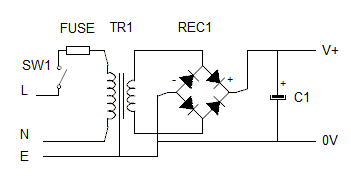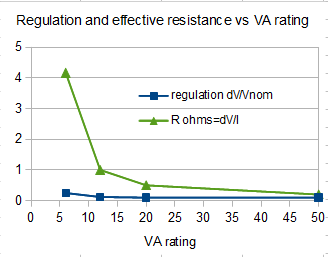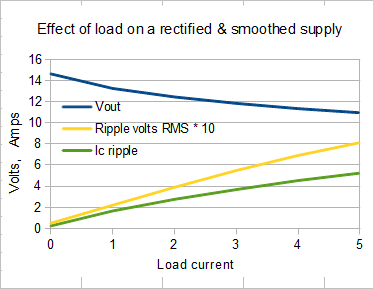# John Errington's tutorial on Power Supply Design

## Load regulation of smoothed full wave rectified supplyWhen we increase the load on this type of supply the output voltage drops.

load regulation = (Voc - Vrated) / Vrated

where Voc = open circuit voltage and

Vrated = output voltage at full rated loadTypical regulation factor for small mains transformers are as follows:

VA rating Regulation Reff ohms
6 0.25 4
12 0.12 1
20 0.1 0.5
50 0.1 0.2
100 0.1 0.1

For a resistive load and sinusoidal waveform the regulation is due to copper losses - resistance in the transformer windings. In power supplies the load is not purely resistive and the waveform is not purely sinusoidal. These factors make accurate calculation of the expected regulation virtually impossible.
(The situation is even worse for half wave rectification.)
For our purposes we can assume the transformer behaves as an ideal sinusoidal voltage source with a series resistance at the secondary.This chart shows the behaviour of output voltage, ripple voltage and ripple current in capacitor with increasing loads.

The components used are as follows:

Tr1 Vpk = 17.0V
VfRec = 2.0V
Rint TR1 = 0.5 ohm
F = 100Hz (2*50Hz)
C1 = 10mF (10,000uF)

If we take 2A as the rated output current the load regulation for this supply would be

%Vlreg= 100*13.6-12.5/12.5 = 8.8%

## Conclusion

When designing a simple linear rectified and smoothed power supply as shown in the figure above the output at full load will be Vrms(full load) = 1.4*Vrms(nominal) - Vf(rectifier) - Vpk-pk(ripple)/2

### Example:

For a 12v 2A supply using a 12V transformer and 4700uF capacitor

Vpk-pk(ripple) = Iload / fC = 2/100*0.0047 = 4.25V

Vrms(full load) = (1.4 * 12V = 16.8V) - 2V - 2.1V = 11.9V

PSUDesigner is a freeware simulator package you can use to check your design.